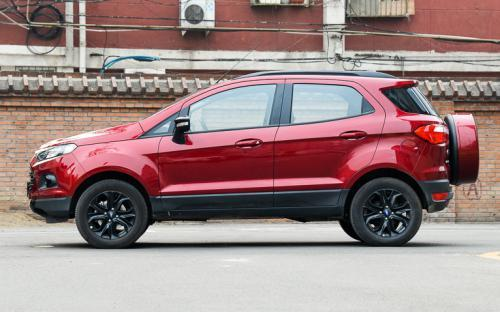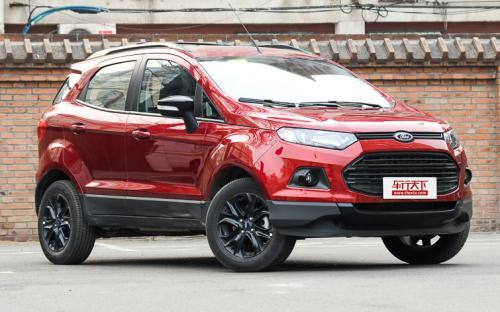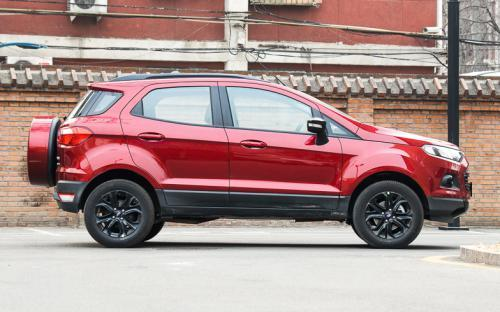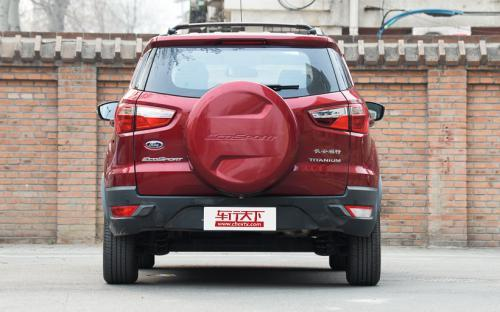### 长安福特 翼搏0 种颜色可选2016款最低售价：12.78 万元起

4280(mm)1785(mm)1658(mm)##### 配置亮点：
• 胎压监测装置

• ISOFIX儿童座椅接口

• 车身稳定控制(ESC/ESP/DSC等)

• 电动天窗

• 定速巡航

• 后倒车雷达

• 真皮座椅

• GPS导航系统

• 氙气大灯

• 后视镜加热

• 提交
2016款 1.5L 自动运动典藏版 (128张)

长安福特 翼搏 绕车实拍• 长安福特 翼搏 在售车型

排量 车型 厂商指导价 本地最低报价 购车工具
1.0L
12.78万
12.78万

1.5L
12.78万
12.78万

长安福特 翼搏 经销商

查看更多 >>

### 长安福特 翼搏 动力加速

翼搏 0-100公里加速时间分布在 0.0-秒 属于 超跑级

动力级别 加速时间 车型

长安福特 翼搏 视频

长安福特 翼搏 新闻资讯

# 叫板奇骏、翼虎 十月钜惠全新途胜只卖良心价

评测 超过8787次关注

各大车企纷纷推出自己的重磅SUV车型。如今，来自北京现代的全新途胜、东风日产的奇骏，以及源自长安福特的翼虎三款SUV，则当仁不让的成为了20万元合资中型SUV市场的...

# 提高工作效率福特翼搏有妙招

汽车导购 超过9268次关注

无论生活，还是工作，高效率成为大家口中的高频词语。但是如何提高效率有是一个难题。搜狐发掘这一难题，联手长安福特翼搏合作发起了一场“拼效率，趣生活”活动，...

# 福特翼搏助阵搜狐时尚盛典

行业动态 超过7625次关注

长安福特翼搏作为一款彰显年青个性的新生代SUV全程赞助了今年的搜狐时尚盛典活动,通过“时尚盛典”活动和年轻群体在时尚态度上产生的强烈共鸣，福特翼搏确立了与都...

# 长安福特翼搏爱冒险 助阵搜狐时尚盛典

行业动态 超过8385次关注

12月23日，时尚又爱冒险的福特家族“鲜肉”长安福特翼搏也将与明星一起现身盛典现场。一直偏爱运动冒险活动的长安福特翼搏，这次是第一次参加时尚盛典，它会如何让...

# 长安福特召回翼搏1.0T 燃油渗漏隐患

碰撞安全 超过5591次关注

日前，长安福特汽车有限公司根据《缺陷汽车产品召回管理条例》的要求，向国家质检总局备案了召回计划，决定自2014年12月20日起，召回2013年5月27日至2014年1月28日...

# 风格各异 福特推出三款翼搏概念车型

进口新车 超过5720次关注

日前，福特在巴西圣保罗车展推出了三款EcoSport（翼搏）概念车型，三款车的外形设计各有特色。

# 长安铃木锋驭竞品分析 长安福特翼搏介绍

车系文章 超过3213次关注

翼搏是合资小型SUV的第二款产品，它的出现直接分走了一大批昂科拉的潜在用户。抛开四大样儿（外观、内饰、空间、动力）不说，光售价区间便足以吸引不少人的眼球。单...

# 内饰材质及配置对比 标致2008配置丰富

车系文章 超过4046次关注

内饰材质及配置、乘坐空间及储物空间对比配置丰富

# 长安福特翼搏C-NCAP碰撞测试实拍解析

碰撞安全 超过5798次关注

2014年2月20日下午，长安福特翼搏1.5L自动尊贵型在中国汽车技术研究中心的新碰撞实验室内，完成了自身的碰撞测试。对于一款非常热销的SUV车型，相信许多消费者对它...

# 1月-4月热销小型SUV盘点 福特翼搏

车系文章 超过2818次关注

福特翼搏2014年1月-4月销量：24798辆

# 合资城市SUV车型盘点 性价比优先

车系文章 超过3039次关注

不仅仅是中国市场，SUV在全球都是强有力的市场增长点。高高的视野、不俗的载物能力以及良好的通过性都是SUV的优势。而合资车型在部分消费者眼中是品质和售后的保障...

# 福特改款翼搏效果图曝光 或于2016年推出

车系文章 超过3125次关注

日前，海外媒体曝光了福特改款翼搏的效果图，新车前脸变化明显，据悉改款翼搏或在2016年上半年推出。

猜你喜欢

﻿
• 快速找车
• 选择品牌
• 选择品牌
• A  奥迪
• A  阿斯顿·马丁
• A  阿尔法·罗密欧
• B  宝沃
• B  布加迪
• B  巴博斯
• B  保时捷
• B  宾利
• B  奔驰
• B  宝马
• B  本田
• B  别克
• B  标致
• B  比亚迪
• B  宝骏
• B  北汽制造
• B  北汽新能源
• B  北汽幻速
• B  北汽威旺
• B  北京汽车
• B  奔腾
• B  北汽绅宝
• C  长安
• C  长安商用
• C  长城
• C  昌河
• D  大众
• D  道奇
• D  DS
• D  东南
• D  东风风神
• D  东风风行
• D  东风小康
• D  东风风度
• D  东风
• F  福特
• F  丰田
• F  菲亚特
• F  法拉利
• F  福田
• F  福迪
• F  福汽启腾
• G  观致
• G  广汽传祺
• G  广汽吉奥
• G  GMC
• H  红旗
• H  汉腾汽车
• H  哈弗
• H  哈飞
• H  海格
• H  海马
• H  华颂
• H  黄海
• H  华泰
• H  恒天
• J  吉利汽车
• J  捷豹
• J  Jeep
• J  江淮
• J  江铃
• J  金杯
• J  九龙
• J  金旅
• K  凯翼
• K  凯迪拉克
• K  克莱斯勒
• K  科尼塞克
• K  卡威
• K  开瑞
• L  路虎
• L  林肯
• L  劳斯莱斯
• L  兰博基尼
• L  雷克萨斯
• L  铃木
• L  雷诺
• L  理念
• L  力帆
• L  莲花汽车
• L  猎豹
• L  路特斯
• L  陆风
• M  马自达
• M  MG
• M  MINI
• M  玛莎拉蒂
• M  摩根
• M  迈凯轮
• N  纳智捷
• O  欧宝
• O  讴歌
• O  欧朗
• Q  奇瑞
• Q  起亚
• Q  启辰
• R  日产
• R  荣威
• R  瑞麒
• S  三菱
• S  斯威汽车
• S  萨博
• S  smart
• S  斯柯达
• S  斯巴鲁
• S  思铭
• S  双龙
• S  上汽大通
• S  双环
• T  特斯拉
• T  腾势
• W  沃尔沃
• W  五菱汽车
• W  五十铃
• W  威兹曼
• W  威麟
• X  现代
• X  雪佛兰
• X  雪铁龙
• X  西雅特
• Y  一汽
• Y  英菲尼迪
• Y  英致
• Y  依维柯
• Y  野马汽车
• Y  永源
• Z  众泰
• Z  中华
• Z  中兴
• Z  知豆
• 选择车系
• 选择车系
• 车型对比
• 选择品牌
• 选择品牌
• A  奥迪
• A  阿斯顿·马丁
• A  阿尔法·罗密欧
• B  宝沃
• B  布加迪
• B  巴博斯
• B  保时捷
• B  宾利
• B  奔驰
• B  宝马
• B  本田
• B  别克
• B  标致
• B  比亚迪
• B  宝骏
• B  北汽制造
• B  北汽新能源
• B  北汽幻速
• B  北汽威旺
• B  北京汽车
• B  奔腾
• B  北汽绅宝
• C  长安
• C  长安商用
• C  长城
• C  昌河
• D  大众
• D  道奇
• D  DS
• D  东南
• D  东风风神
• D  东风风行
• D  东风小康
• D  东风风度
• D  东风
• F  福特
• F  丰田
• F  菲亚特
• F  法拉利
• F  福田
• F  福迪
• F  福汽启腾
• G  观致
• G  广汽传祺
• G  广汽吉奥
• G  GMC
• H  红旗
• H  汉腾汽车
• H  哈弗
• H  哈飞
• H  海格
• H  海马
• H  华颂
• H  黄海
• H  华泰
• H  恒天
• J  吉利汽车
• J  捷豹
• J  Jeep
• J  江淮
• J  江铃
• J  金杯
• J  九龙
• J  金旅
• K  凯翼
• K  凯迪拉克
• K  克莱斯勒
• K  科尼塞克
• K  卡威
• K  开瑞
• L  路虎
• L  林肯
• L  劳斯莱斯
• L  兰博基尼
• L  雷克萨斯
• L  铃木
• L  雷诺
• L  理念
• L  力帆
• L  莲花汽车
• L  猎豹
• L  路特斯
• L  陆风
• M  马自达
• M  MG
• M  MINI
• M  玛莎拉蒂
• M  摩根
• M  迈凯轮
• N  纳智捷
• O  欧宝
• O  讴歌
• O  欧朗
• Q  奇瑞
• Q  起亚
• Q  启辰
• R  日产
• R  荣威
• R  瑞麒
• S  三菱
• S  斯威汽车
• S  萨博
• S  smart
• S  斯柯达
• S  斯巴鲁
• S  思铭
• S  双龙
• S  上汽大通
• S  双环
• T  特斯拉
• T  腾势
• W  沃尔沃
• W  五菱汽车
• W  五十铃
• W  威兹曼
• W  威麟
• X  现代
• X  雪佛兰
• X  雪铁龙
• X  西雅特
• Y  一汽
• Y  英菲尼迪
• Y  英致
• Y  依维柯
• Y  野马汽车
• Y  永源
• Z  众泰
• Z  中华
• Z  中兴
• Z  知豆
• 选择车系
• 选择车系
• 选择车型
• 选择车型
• 意见反馈